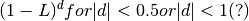# statsmodels.tsa.arima_process.lpol_fiar¶

`statsmodels.tsa.arima_process.``lpol_fiar`(d, n=20)[source]

AR representation of fractional integrationParameters: d : float fractional power n : int number of terms to calculate, including lag zero ar : array coefficients of lag polynomial Notes: first coefficient is 1, negative signs except for first term, ar(L)*x_t